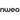# Fun Place Value Chart [+ Teaching Tips and 8 Activities]

Maria Kampen

All PostsWe use big numbers everyday, whether we’re counting money, weighing objects or planning a trip.A place value chart is an excellent tool for helping students make sense of large numbers. Place value is a foundational math concept, necessary for addition, subtraction, exponents, long division, fractions and almost every other math operation. In this article:

## Printable place value chart

Many learners understand place value best when they work with the numbers themselves. A place value chart gives students the chance to see the value of digits, numbers and decimals in our base ten number system.Here are three charts to use with your students as they learn place value! Plus, we've made it easy for you to choose between colorful and printer-friendly options.

### Blank place value chart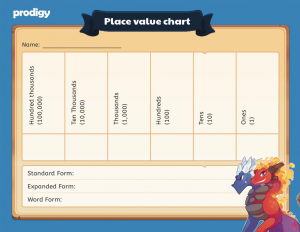Click to download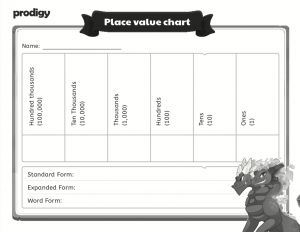Click to downloadStudents can use a blank place value chart to take numbers and easily assign a place value to each digit. For added practice, use a place value chart with opportunities for students to write out the number in standard, expanded and word form.Want to make a reusable place value chart? Download, print and laminate these sheets. Students can use dry erase markers to write their numbers, then wipe them off and start again!

### Place value chart to billions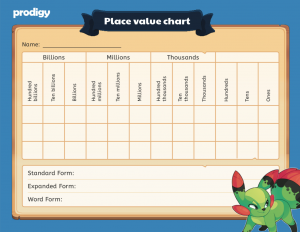Click to download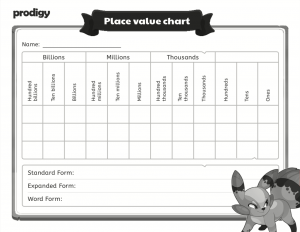Click to downloadThrill and amaze students when you teach them to make gigantic numbers using a place value chart. This chart also includes spaces for students to write out their answers in standard, expanded and word form. Have students work with famous numbers, like:
• 252,088 miles — The furthest distance between Earth and the moon
• 7,581,154,200 — The world population in June 2019, as estimated by the US Census Bureau
• 8,398,748 — The population of New York City as of July 1st, 2018
• 35,800,000 miles — The distance between Earth and Mars at the closest point in Mars’s orbit
They’ll learn about science and geography, while also practicing their place value skills! Place value charts also teach students the difference between place value and period value. Place value is the value of an individual digit, while period value refers to groups of three places separated by commas.

### Decimal place value chart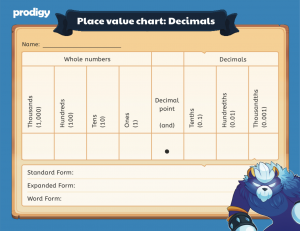Click to download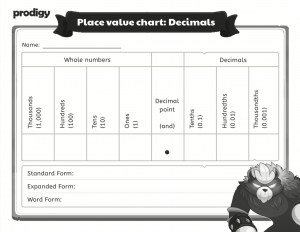Click to downloadInstead of moving to the left of the decimal point and multiplying by ten, divide by ten or multiply by ⅒ to determine decimal place value. A place value chart with decimals can help students see how numbers become smaller when they move to the right of the decimal place.

## Use Prodigy to teach place value!

Want so much more than just a place value chart? Prodigy is a no-cost, curriculum-aligned math platform that makes learning math fun! Students will explore an exciting world filled with adventure, where success depends on correctly answering skill-building math questions.Teachers have access to a powerful dashboard where they can see where students are struggling or excelling and control the questions students receive in-game.Using Prodigy in your classroom promotes a daily math practice and keeps students engaged with in-class content. With more than 1,400 skills across three DOK levels, you’ll be able to align in-game content with anything you’re teaching in the classroom — including place value!Learn more about using Prodigy in your classroom: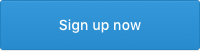## What is place value?

View this post on Instagram

A post shared by Amy Groesbeck (@theamygroesbeck) on

Place value is the value of a digit as determined by its position in a number.  Students learn place value to understand that every number is made up of digits. These digits -- 0-9 -- have different values depending on their place in the number. Up until this point, students have been learning numbers as a continuous sequence. They don’t necessarily understand the base ten system or realize numbers are made up of other numbers.

### Whole numbers

The best way to start teaching students about place value is through hands-on examples. Using pebbles, beans or other small objects, ask the students to count out 17. Explain to students they can divide 17 into a group of ten and a group of seven. In 17, the one represents the group of ten and the seven represents seven ones.Do this a few more times, with a larger number each time. (For example, move from 17 to 24, then to 43). Use a place value chart to explain that each digit in the tens column represents groups of ten. For example, a four in the tens column would represent 40, which is four groups of ten. This is a good time to start teaching some of the vocabulary around numbers and place value students will need to know.Standard form: The number, written as usual.
Example: 3,453
Written form: The number written out using words instead of digits.
Example: three thousand four hundred fifty three
Expanded form: The number written out as the product of the digit multiplied by the place value.
Example: (3 x 1000) + (4 x 100) + (5 x 10) + (3 x 1)
Expanded form is an excellent way to illustrate place value with students who have already learned the basics of multiplication. With each place further to the left, the value of the digit is multiplied by ten.  Students should also be able to use the less than (<), greater than (>) or equal to (=) signs to express the relationship between two numbers as a sentence:

3,453 > 2,232

“3,453 is greater than 2,232.”

Do your students struggle with the difference between these signs or with expressing which number is greater? Try these tricks to help them remember:
• The Hungry Alligator — The greater than (>) or less than (<) signs are an alligator’s mouth, and the number on either side represents a number of fish. The alligator always wants to eat the largest number of fish, so the mouth is always open towards the larger number.
• Read out loud — Students aren’t used to this kind of mathematical language. Make sure students can express this as a sentence in their writing and reading, and they understand “greater” refers to the amount, not the quality, of the number.
• Teach students to look at the greatest place value first — Students should realize the digit furthest to the left has the greatest place value. If those are the same, teach students to move towards the right until they find a number that’s greater.
Students can also use a place value chart to compare numbers.Other important symbols include:
Greater than or equal to
Less than or equal to
Does not equal

### Decimals

Instead of getting larger to the left, decimals move to the right and get progressively smaller.  Place value in whole numbers is determined by multiples of ten, but the place value of decimals is based on multiplying by ⅒. There are a few terms students should understand before diving into decimal place value:
• Decimal point — The point that marks where the whole number ends and the decimal begins. When written out in word form, a decimal point is expressed with “and.
• Tenth — ⅒ of a whole, the first place value directly to the right of the decimal.
• Hundredth — ¹⁄₁₀₀ of a whole, the second place value to the right of the decimal.
As a rule, numbers to the right of the decimal end with “th”: hundredth, thousandth, ten thousandth, and so on. When you’re working with decimal place value charts, ask students why they might need to use numbers with decimals. Some common answers could include:
• Money
• Timing a race
• Measuring objects
Ask students what the decimal places in those answers represent. Students have already worked with decimals if they’ve worked with money and time, but they might not realize decimals are connected to place value and represent numbers less than one.Using a place value chart with decimals, challenge students to write out numbers with decimals in standard and word form.

28.18

Twenty eight and eighteen hundredths

Instead of multiplying by decimals to expand the decimal place, decimals in expanded form can be more accurately represented when we multiply the digit by the appropriate fraction. Since decimals are numbers less than one, each place value can be represented as a fraction.

0.1 = ⅒ = one tenth

0.01 = ¹⁄₁₀₀ = one hundredth

0.001 = ¹⁄₁₀₀₀ = one thousandth

The decimal point in the example above can be expressed as 18 hundredths, or ¹⁸⁄₁₀₀.This means 28.18 could also be expressed as:

(2 x 10) + (8 x 1) + (1 x ⅒) + (8 x ¹⁄₁₀₀) = 28.18

## Activities for teaching place value

### 1. Number walkTake learning out of the classroom and onto the sidewalk with this engaging activity. In your school’s neighbourhood, take students out for a walk and write down any numbers you see on traffic signs, houses or billboards.When you get back to the classroom, talk about which numbers are bigger and smaller. Ask students to group number using common factors, including:
• All the numbers with four digits
• All the numbers with a zero in the ones place
• All the numbers with a three in the tens place
Use a blank place value chart to analyze the numbers with your students and write them in standard, expanded and word form.

### 2. Bingo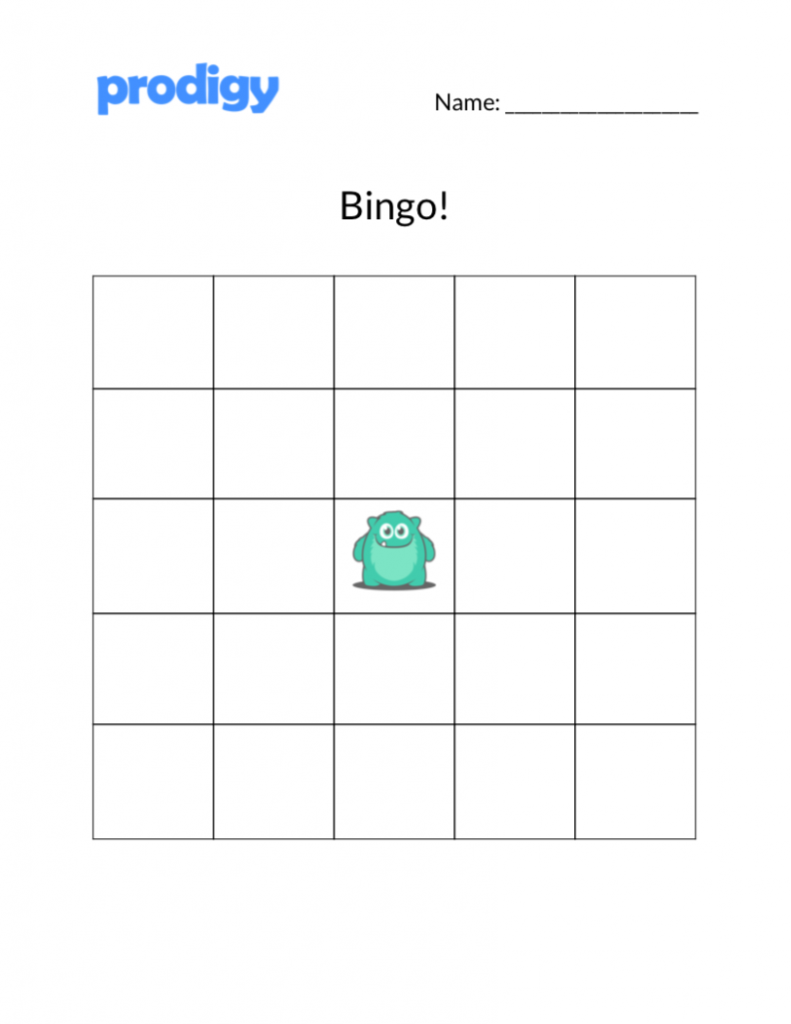Click to downloadBingo is an easy way to introduce gamification into the math classroom for a number of concepts, including place value.Hand out bingo cards to your students with two-, three- or four-digit numbers in each square. You can also ask students to fill in their bingo cards with numbers and swap sheets with a partner.Call out digits and place value for students using sentences like, “I’m looking for a 5 in the tens place,” or “I’m looking for a number with a 300 in it.” The first student to have a full row, either up, down, across or diagonally, gets to call out the next number.

### 3. Abacus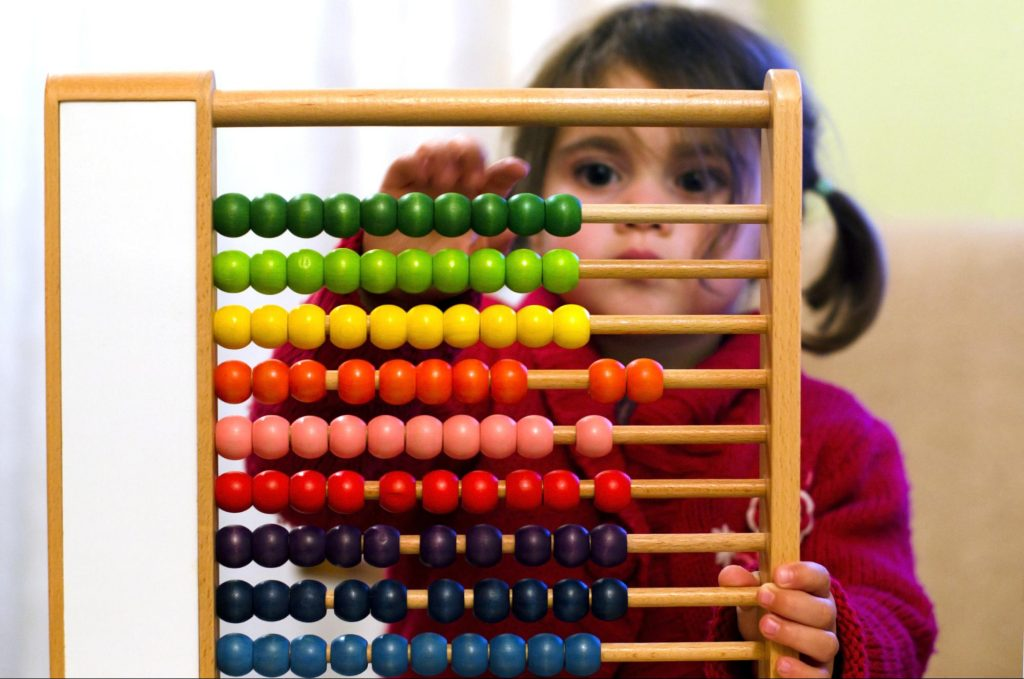For millennia, people around the world have used different types of abacuses to calculate addition, subtraction and even more complicated equations that include square roots and fractions.Use a standard abacus with several rows and ten beads on each row. Each level represents a place value, starting at the top with ones, tens, hundreds and thousands going down.Start with smaller number. For 18, students can count out ten beads from the ones column, then move over a bead in the tens column to represent those ten beads. Finally, students can count out eight beads for the ones column. This helps students understand place value as multiples of ten. To make a larger number like 281, students can take two beads from the hundreds row, eight beads from the tens row, and one bead from the ones row.

### 4. Money

There’s a good chance your students have already identified money as being one practical application of place value, both for whole numbers and decimals. Money encourages abstract thinking. With whole numbers, students can easily use one, 10 and 100 dollar bills to understand how digits can represent large values.

|

Students can also learn how to work with decimals using dimes and pennies. Explain that one dime is worth one-tenth of a dollar. Link money back to earlier lessons on decimal place value to help students recognize decimals as parts of the whole numbers they’re used to working with. As a fun activity, divide students into groups and give each one three piles of one dollar bills, ten dollar bills, dimes and pennies. Using a place value chart, students can put the piles in order from least to greatest, and write out the number in standard, expanded and word form.

### 5. Number storiesInstead of just writing out numbers on a place value chart, give students the chance to think about place value differently with number stories.Give students a sentence with digits and the place value, but not in the right order. Challenge them to write the number 821 with the clue, “I’m thinking of a number with a one in the ones column, an eight in the hundreds place, and 20.”Once students have the story, they can practice writing the number in expanded, word and standard form. Use a place value chart to help keep larger numbers straight.

### 6. Manipulatives

Tried and true, manipulatives are an excellent way for hands-on learners to understand more abstract concepts like place value.

|

There are two types of manipulatives you can use to teach place value:
• Literal manipulatives such as base ten blocks, beans or marbles represent one unit as equaling one number. With place value, literal representation can help students see exactly how many groups of ten or a hundred are in a number.
• Representative manipulatives are objects like counting chips or colored paper, where each color represents ones, tens, hundreds or thousands. These manipulatives help students understand how a single object or digit can represent a very large number.
Use what’s most accessible in your classroom to reinforce the concept of place value with your students. After they’ve made the number with manipulatives, students can use a place value chart to write the number in its different forms.

### 7. Dice roll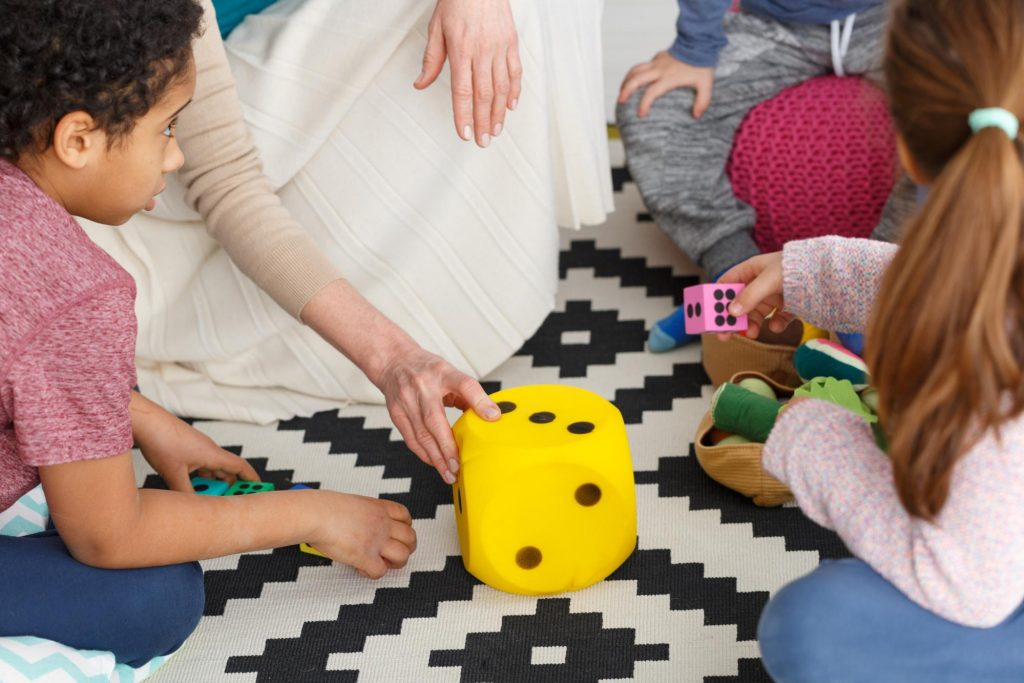Challenge your students to beat the teacher with this fun classroom game! Write a number on the board and hand out dice to each of your students. Students can roll their dice two or three times and use the digits from each roll to create a number greater than, less than or equal to the number on the board. Manipulating the digits gives students the opportunity to think about building place value and the relationship between numbers. Give the student with the largest, smallest or closest number the chance to write a number on the board themselves. For an added challenge, have students use a place value chart to write out their number in standard, expanded and word form.

## When to use a place value chart in your classroom

Place value is a foundational math concepts your students need to understand clearly in order to move on to more difficult units. Use a place value chart in your classroom to run some of the activities above, or as a tool for students to use as they’re completing their work. When students understand how to build bigger and bigger numbers, there’s no limit to what they’ll be able to learn!
Prodigy is a no cost, adaptive math platform that's loved by over a million teachers and 50 million students. Log in or sign up today to see what it can do in your classroom!•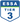•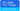•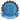•